# Rajasthan Board Question Papers for Class 12th Maths 2019 With Solutions In PDF

Rajasthan Class 12 Maths 2019 question paper with solutions are provided here in a downloadable pdf format and also in the text, so that the students are aware of the maths marking scheme. Along with the solutions, Maths question paper 2019 Class 12 is also added here for reference. Students can solve the RBSE Class 12 Maths Previous Year Question Paper 2019 for practise and then refer to the solutions to gauge their exam preparations.

RBSE 12th Students will be able to access all the RBSE 12th previous year Maths question papers. The solutions provided by BYJU’S will encourage the students to practise well and score high marks in the upcoming RBSE board examination. It can be downloaded easily and in a shorter period of time without any hassle.

Solving 2019 Maths question papers and also the previous year papers for Class 12 will assist the student in analysing and evaluating their preparation in detail.

### QUESTION PAPER CODE 1310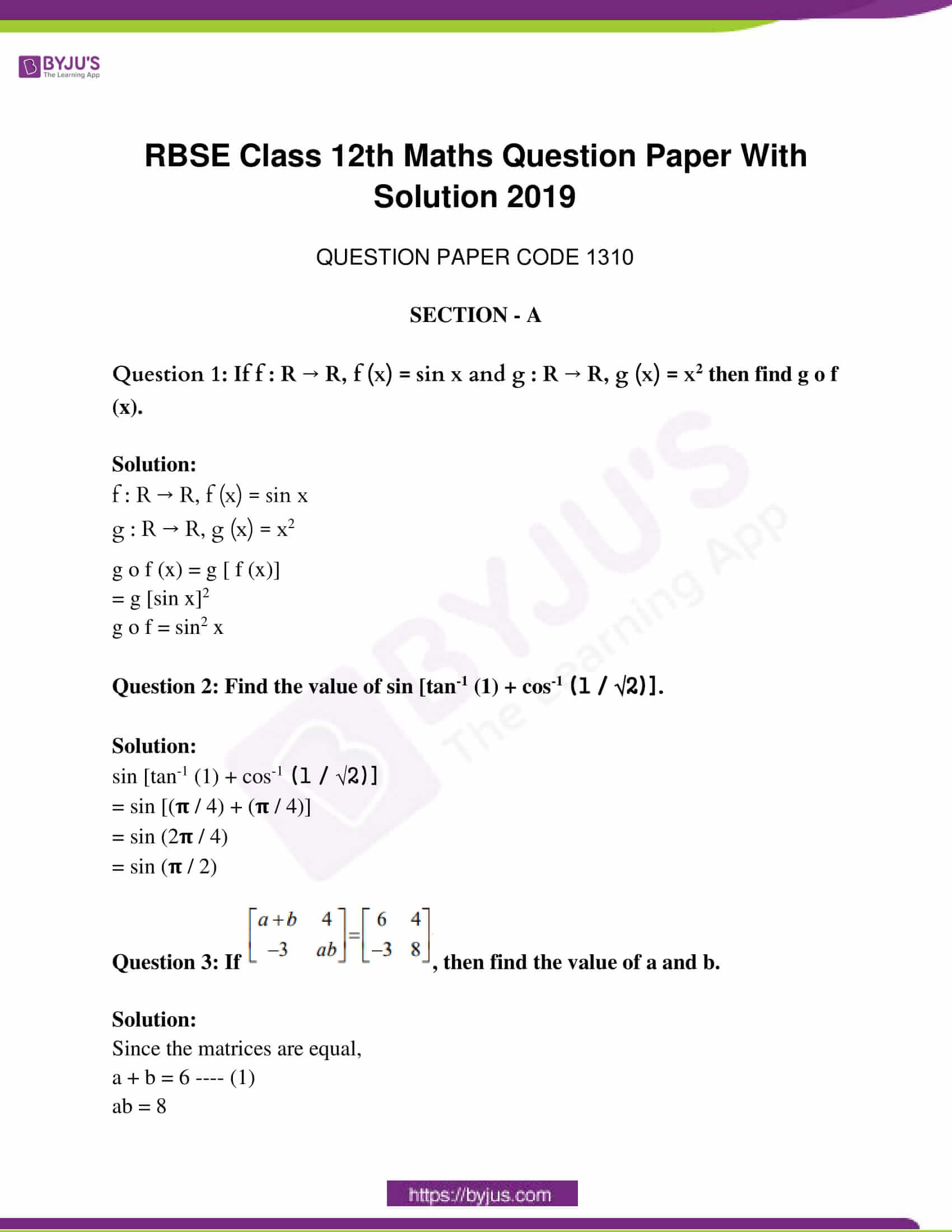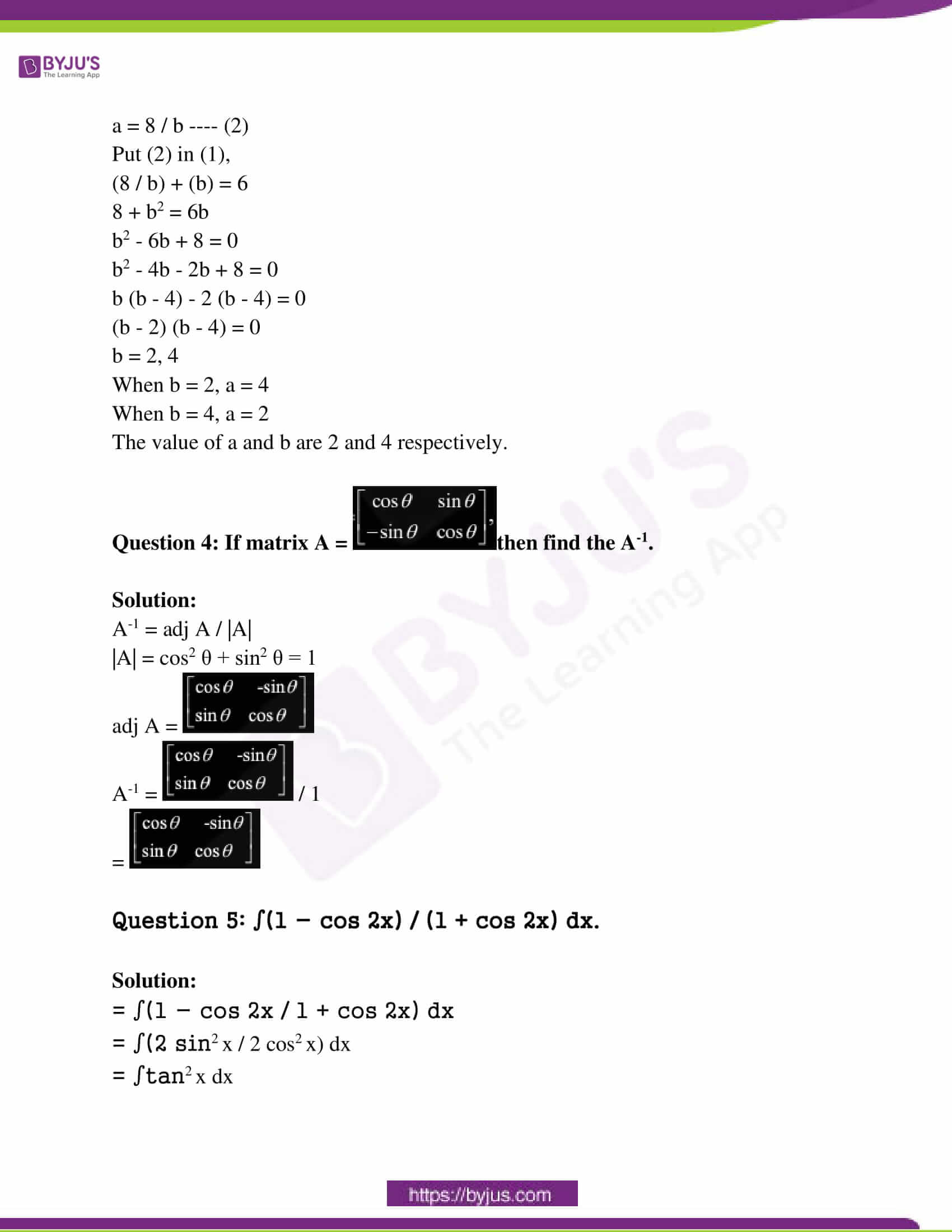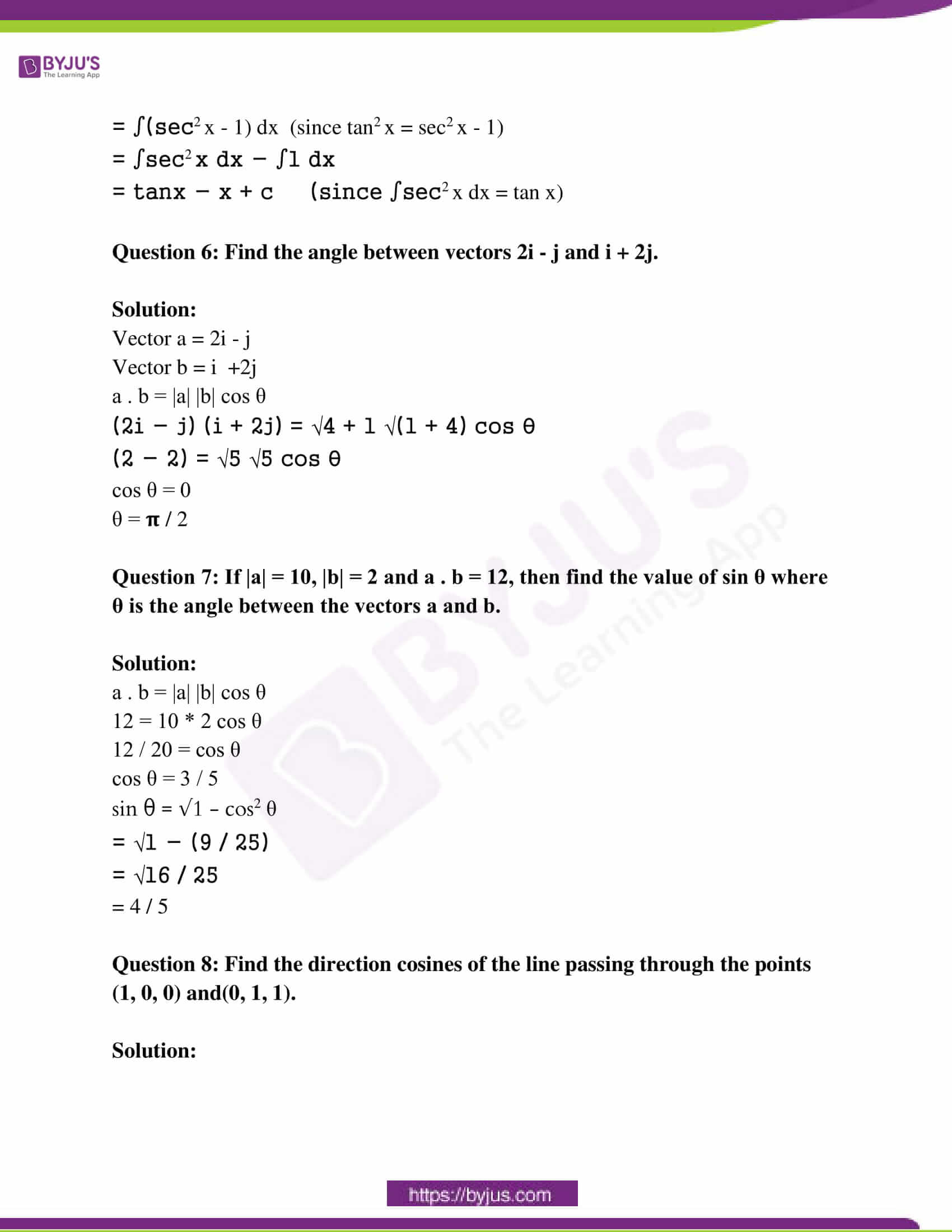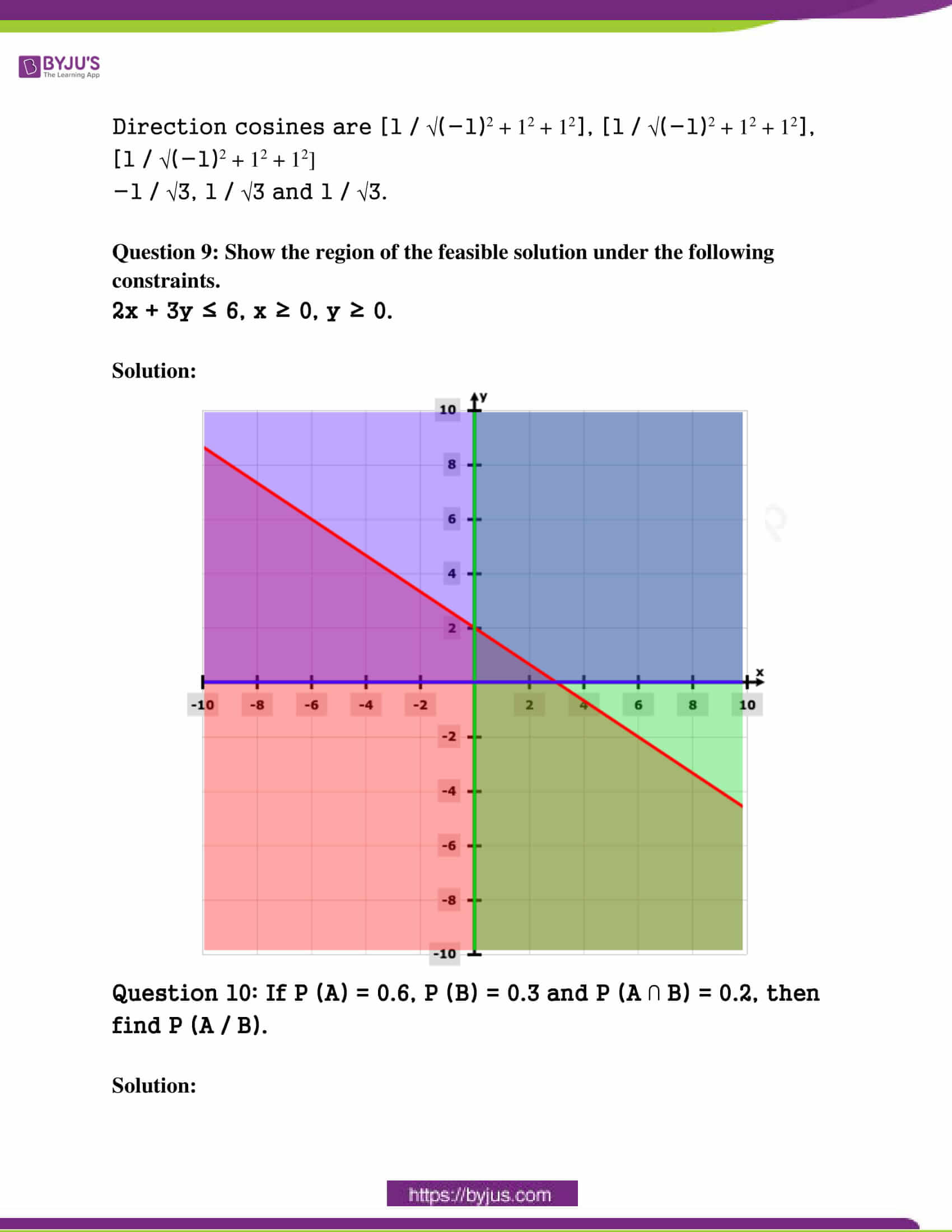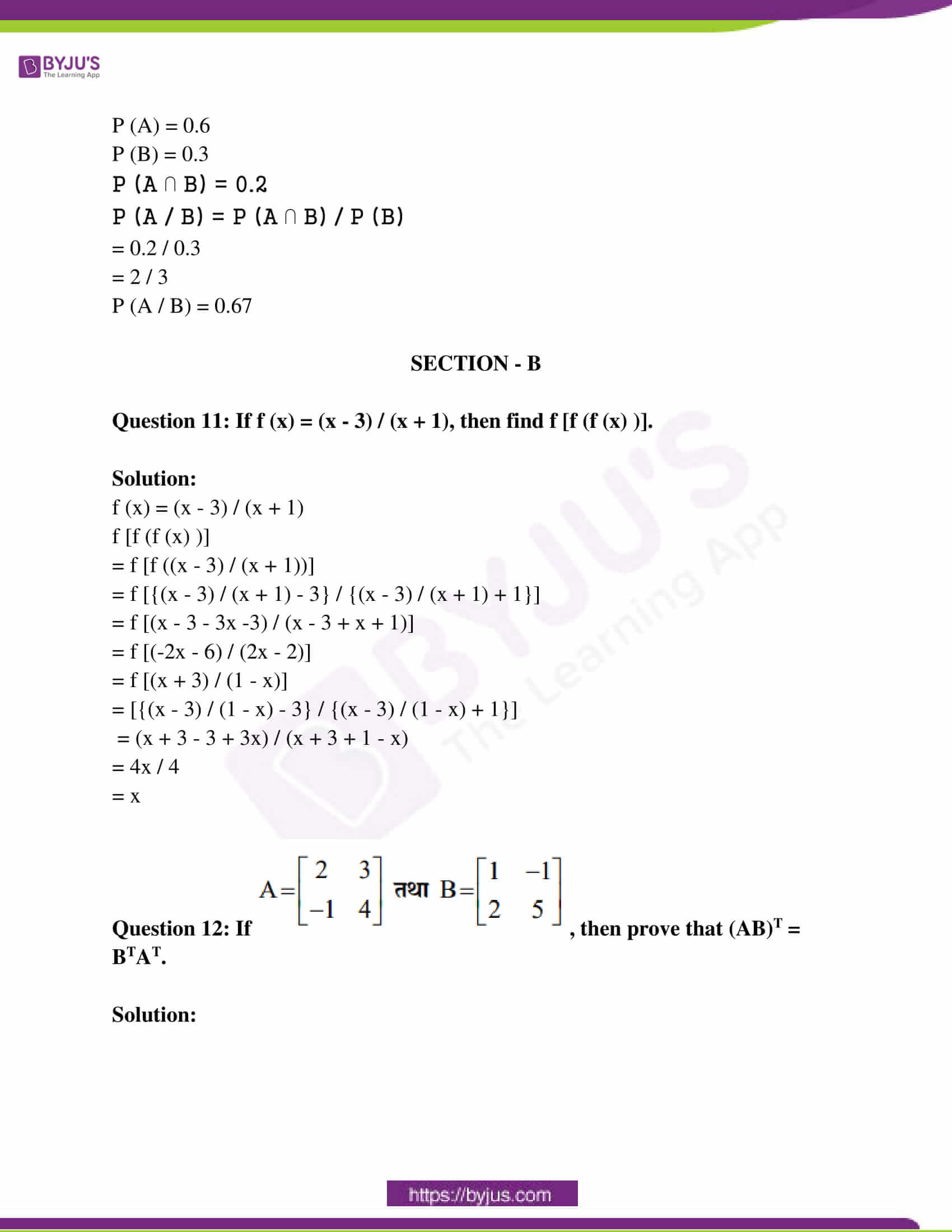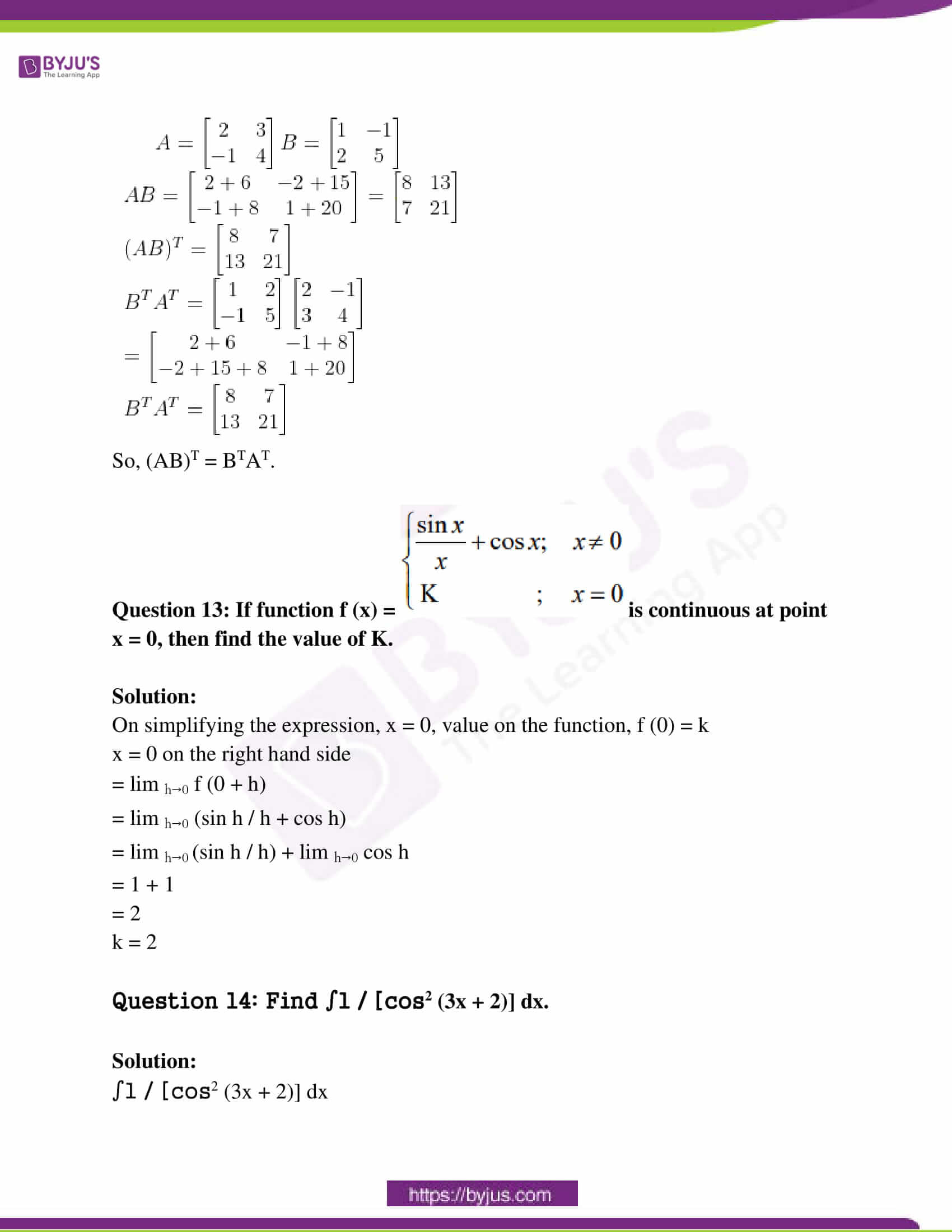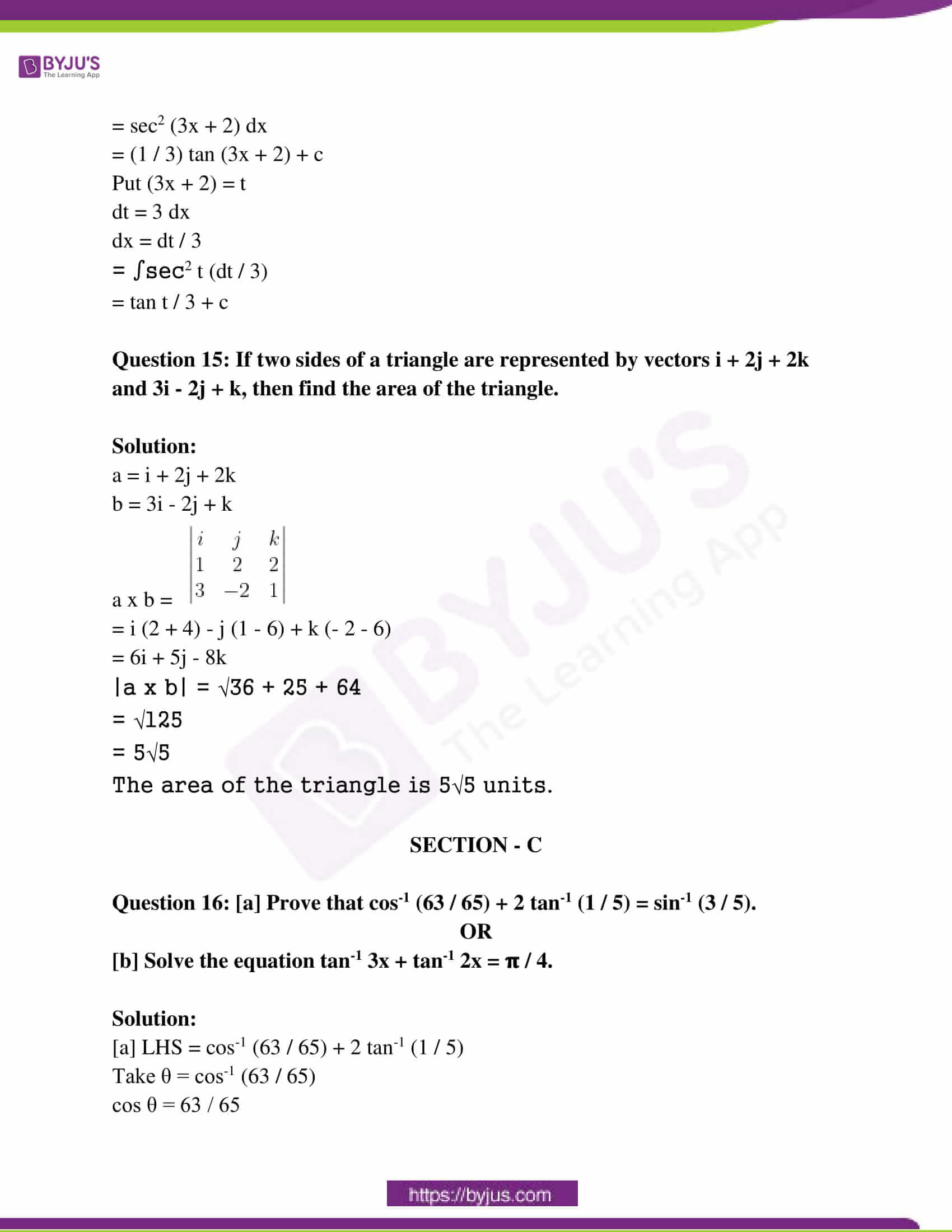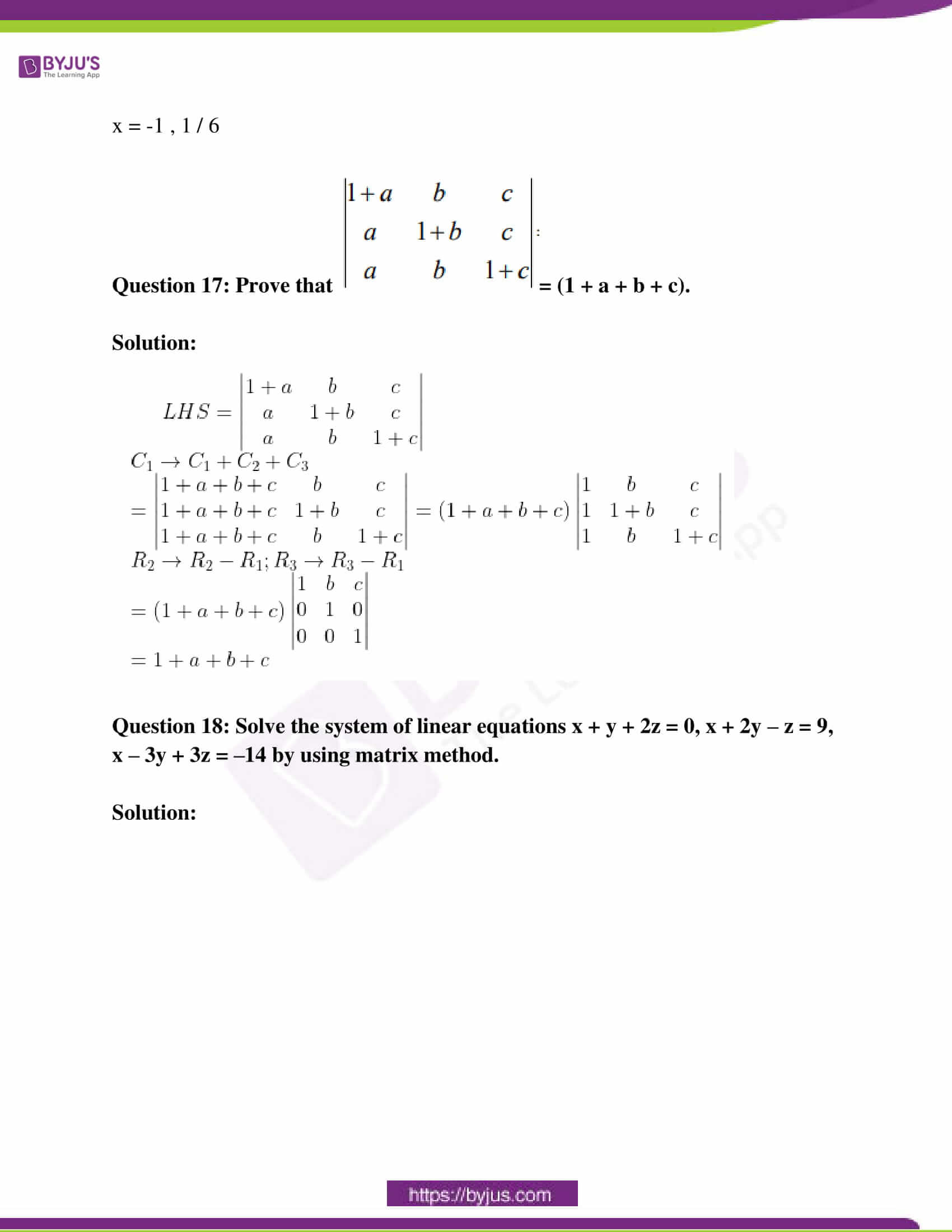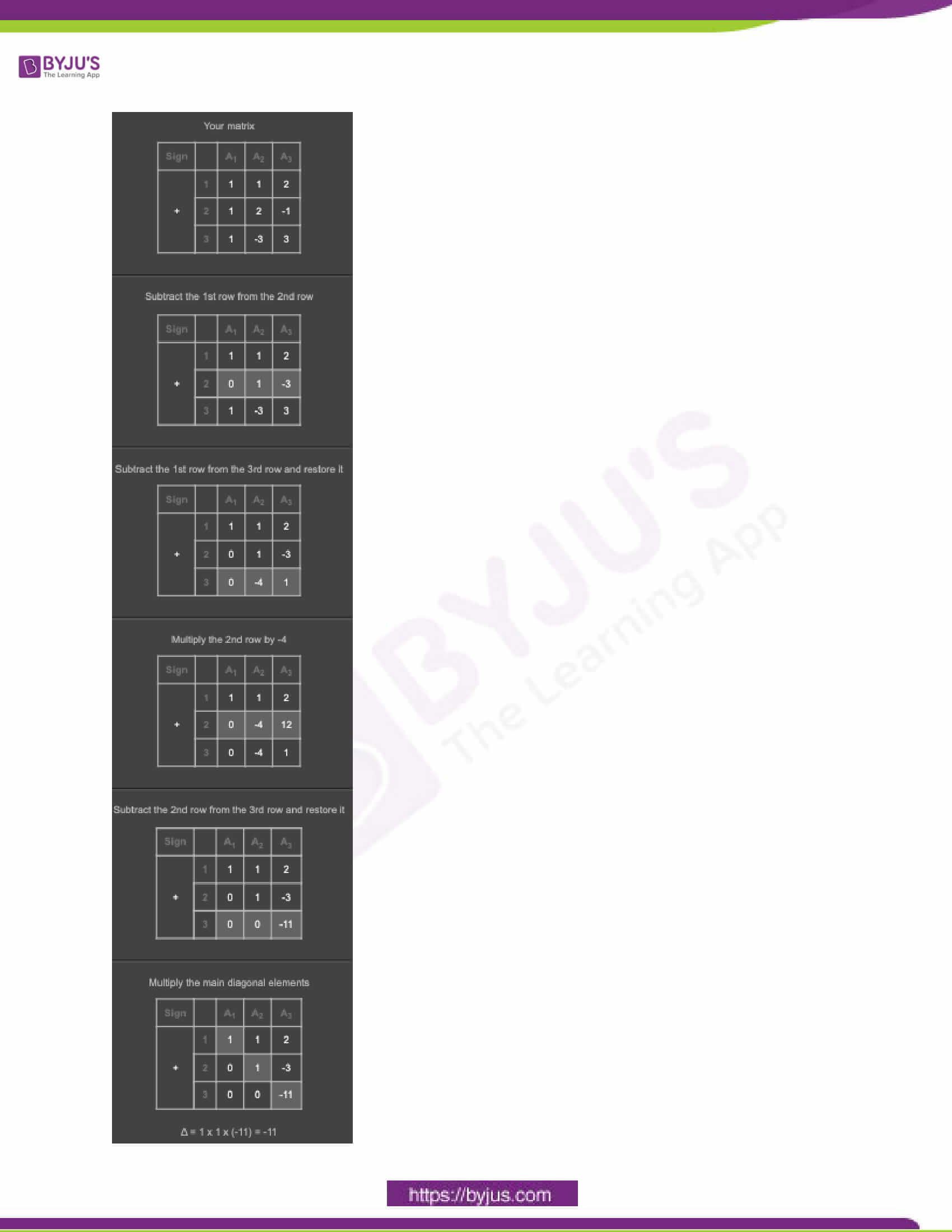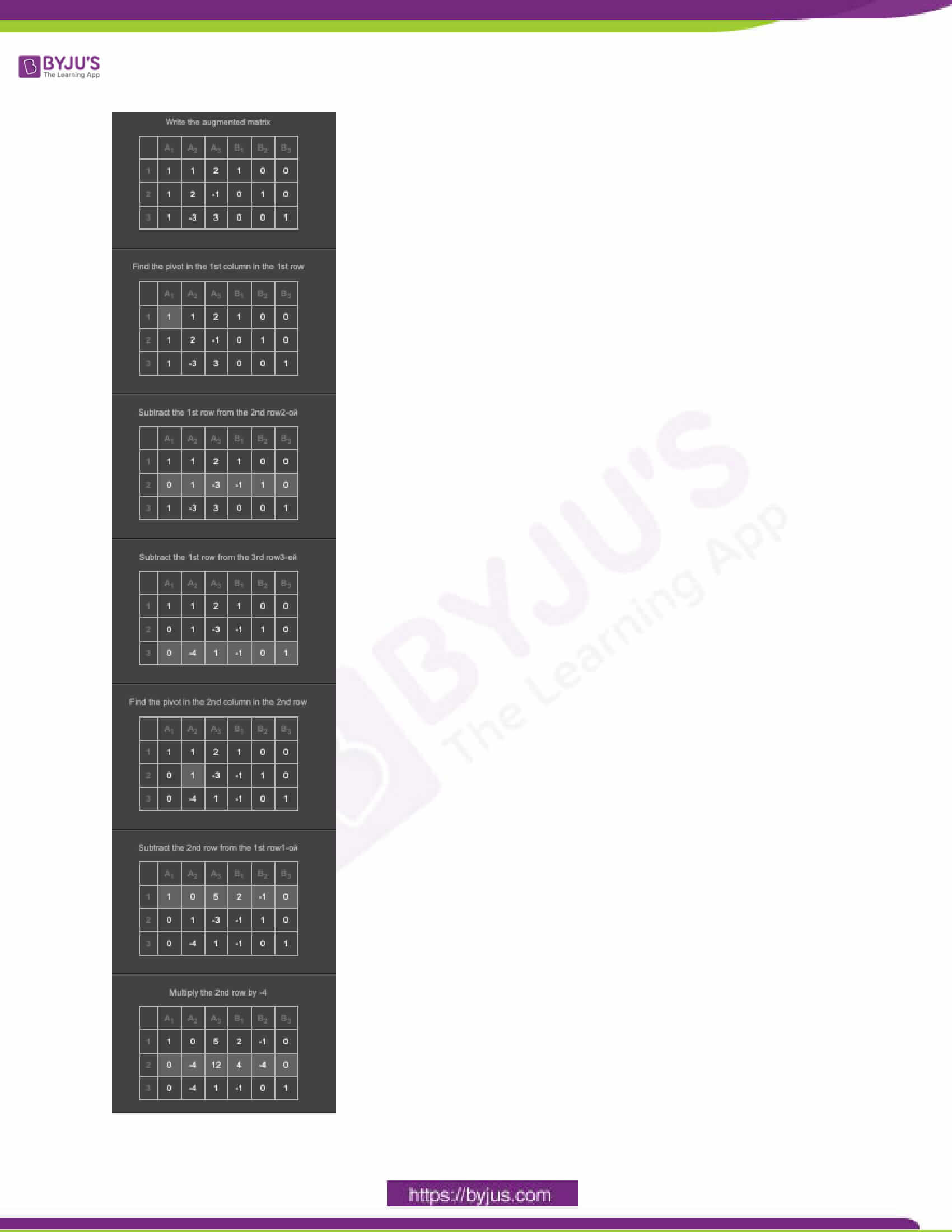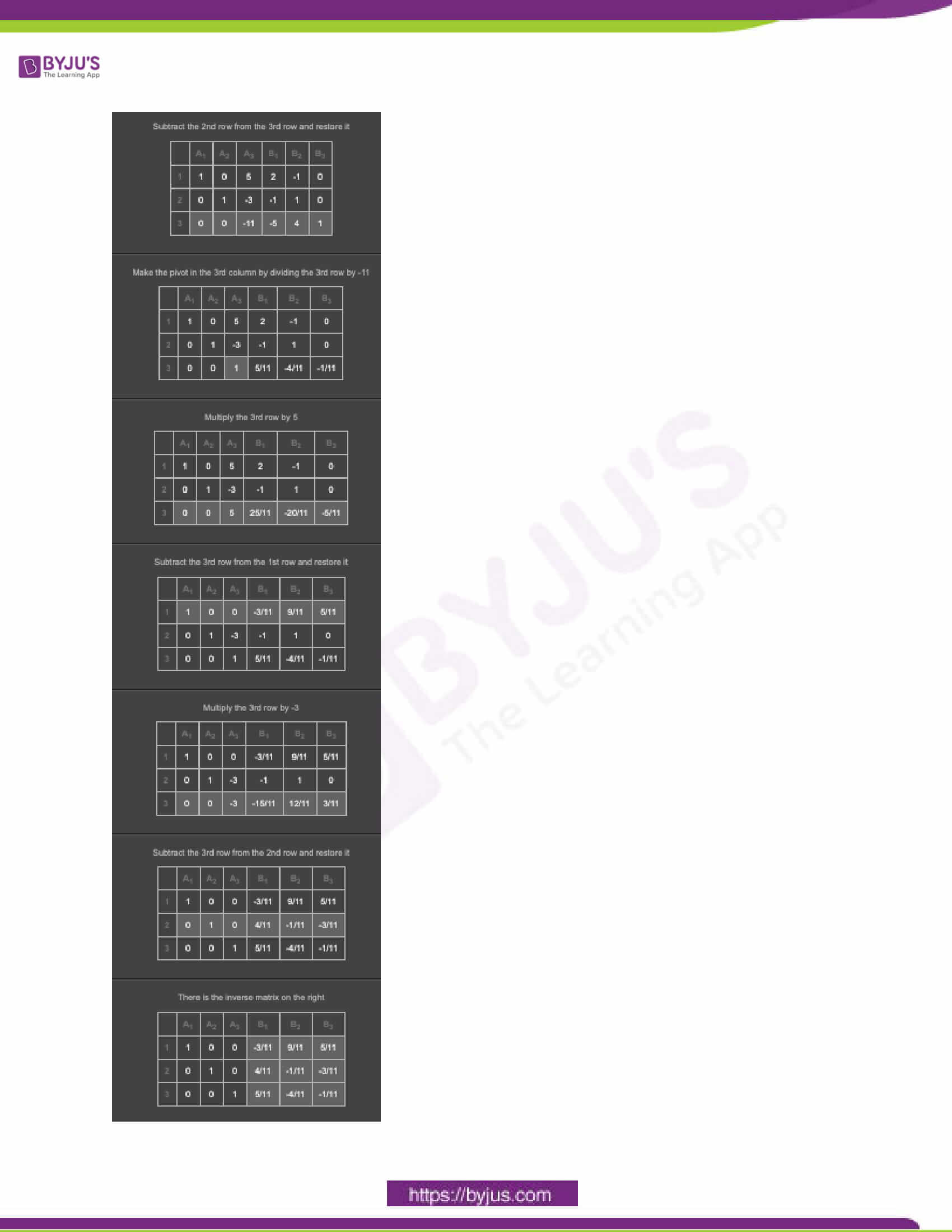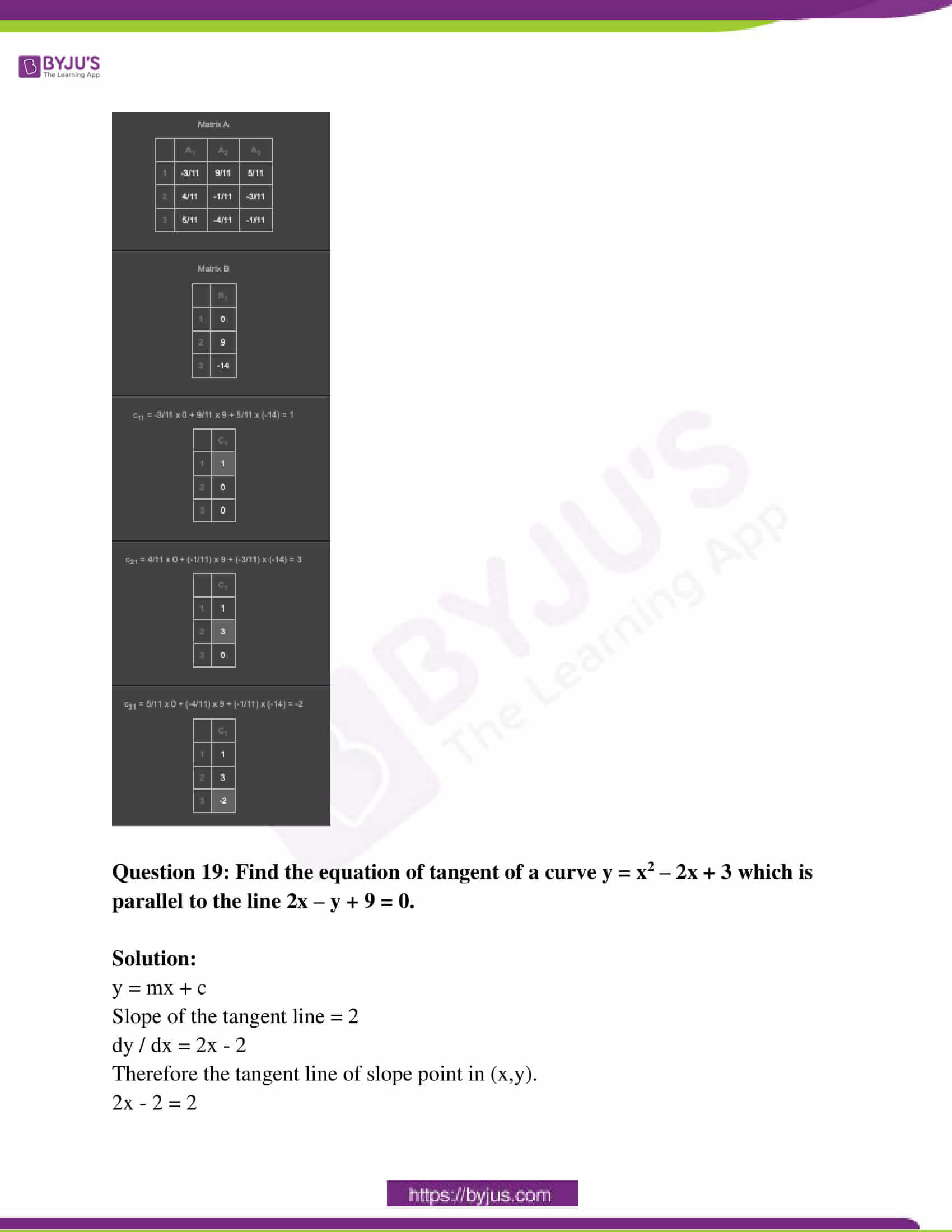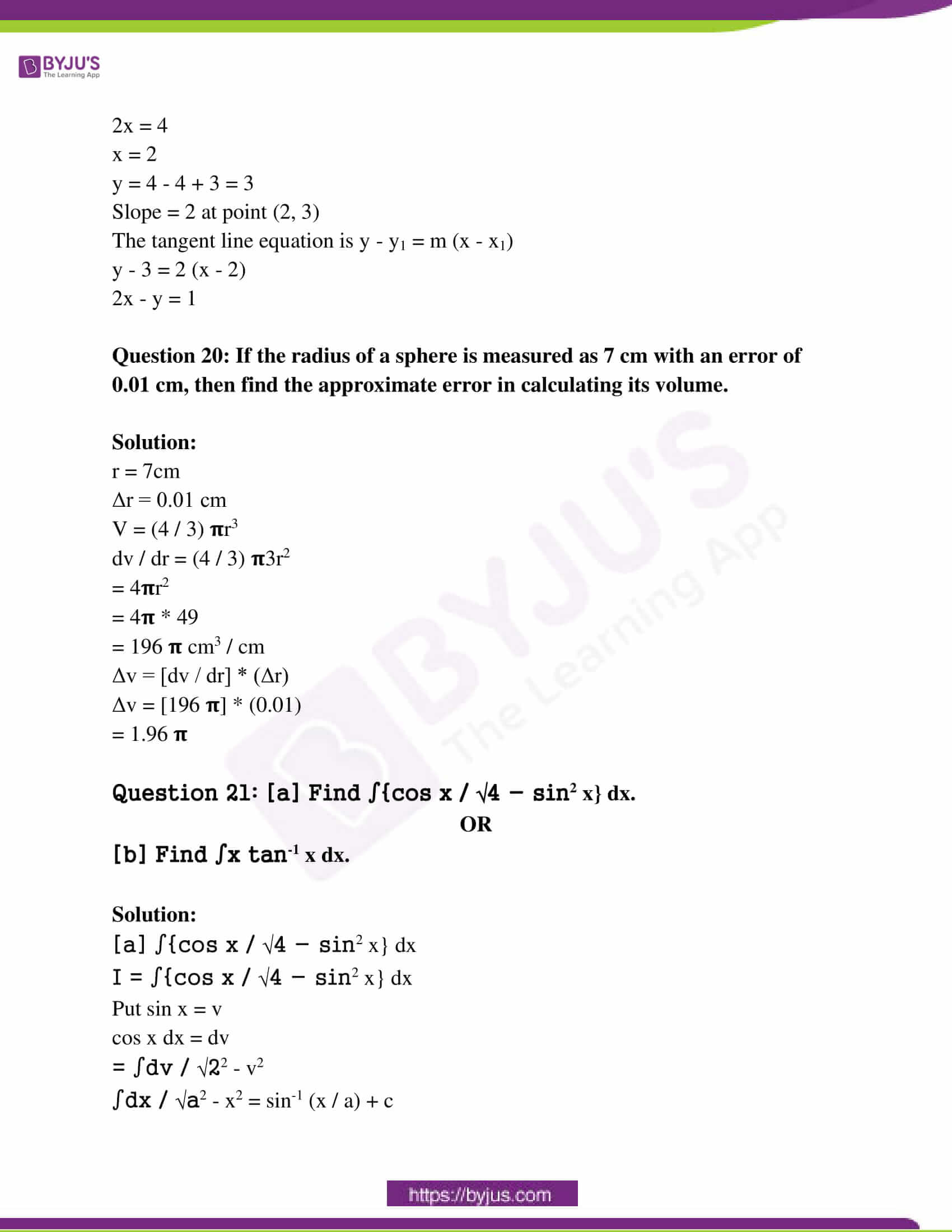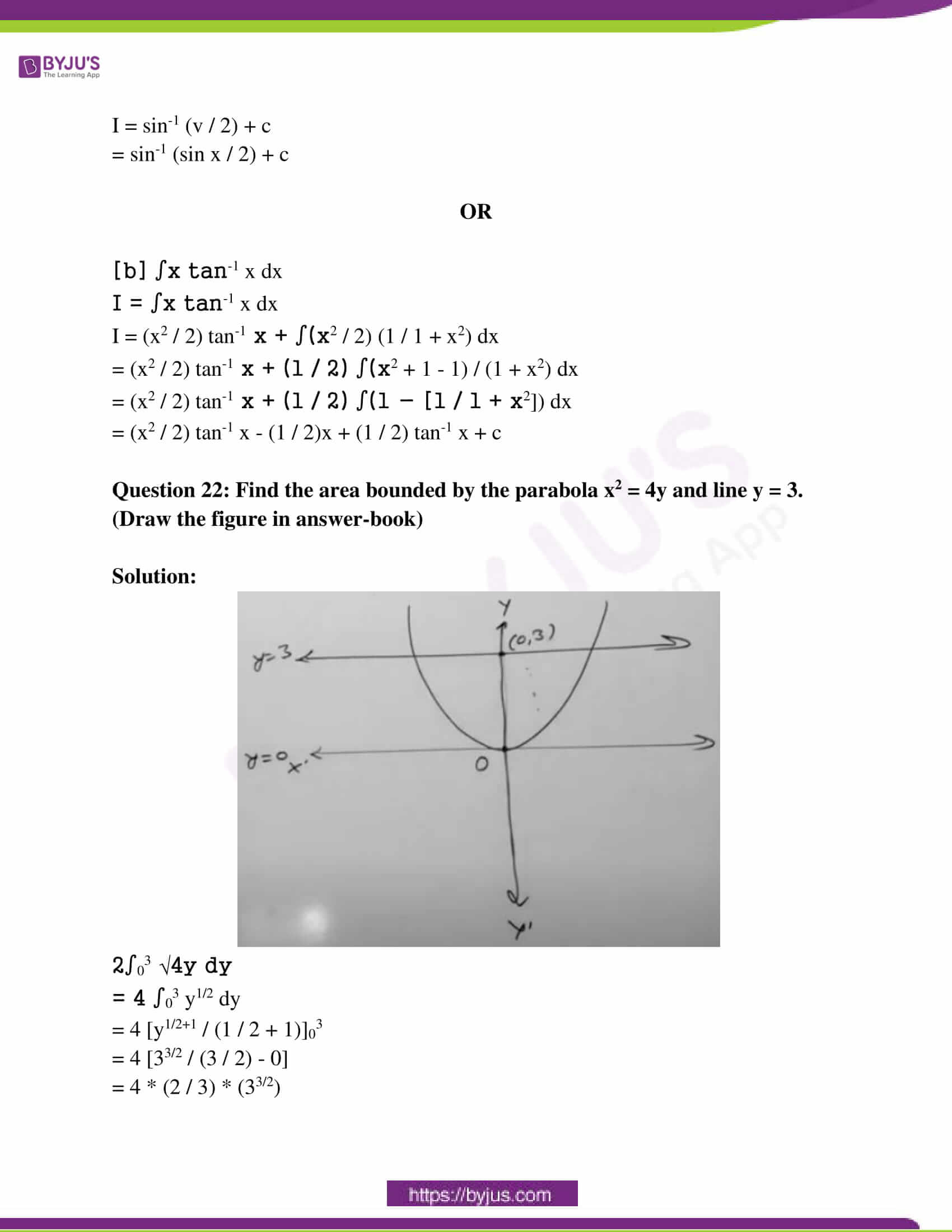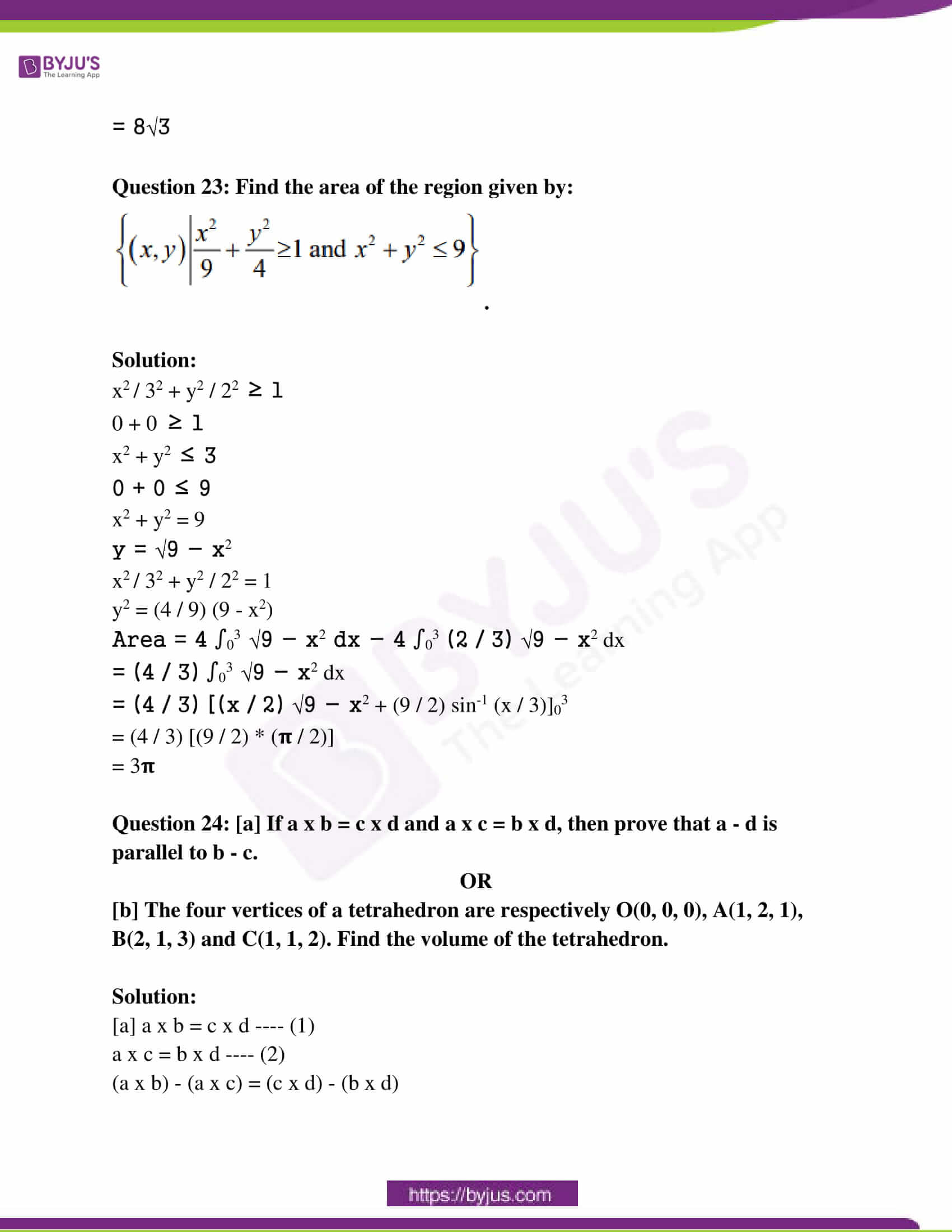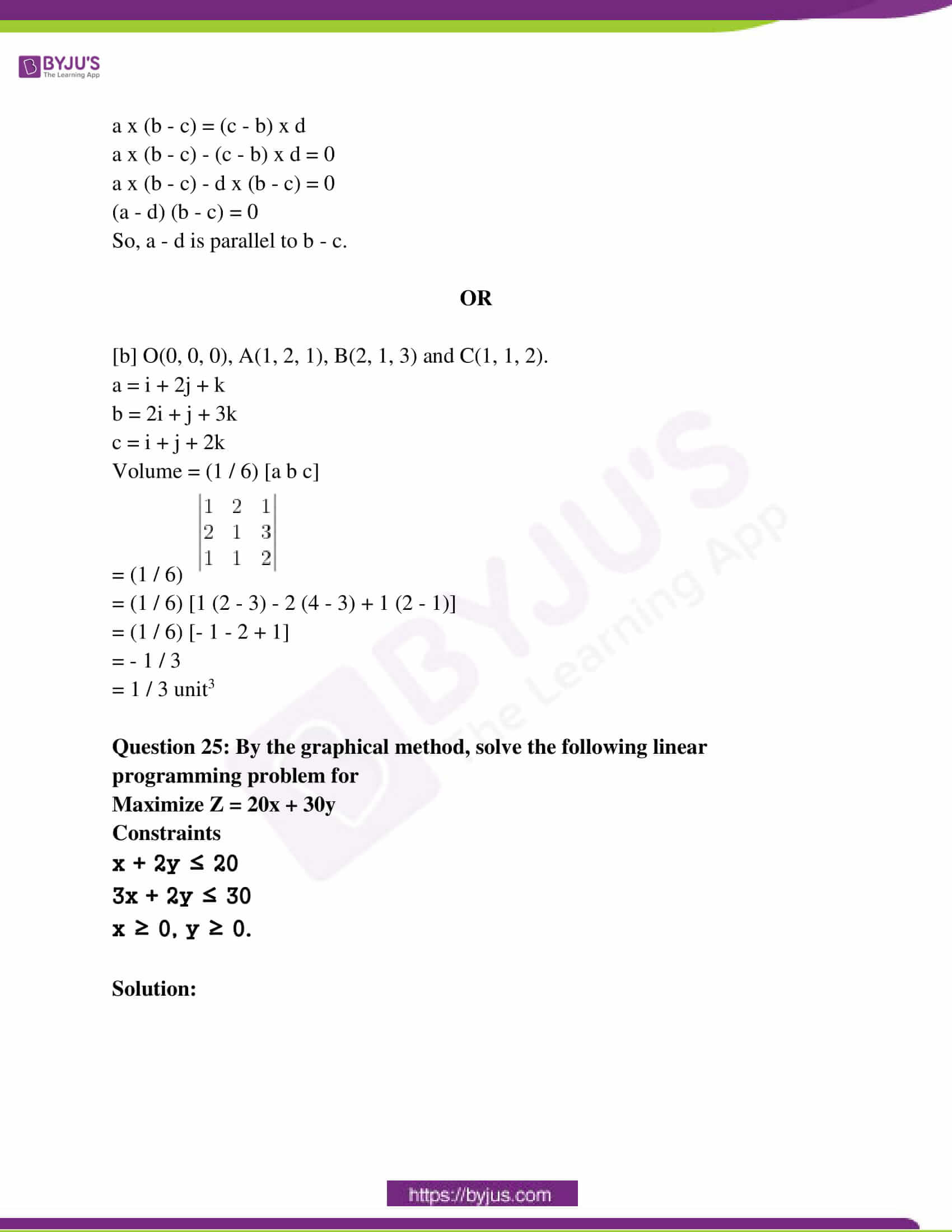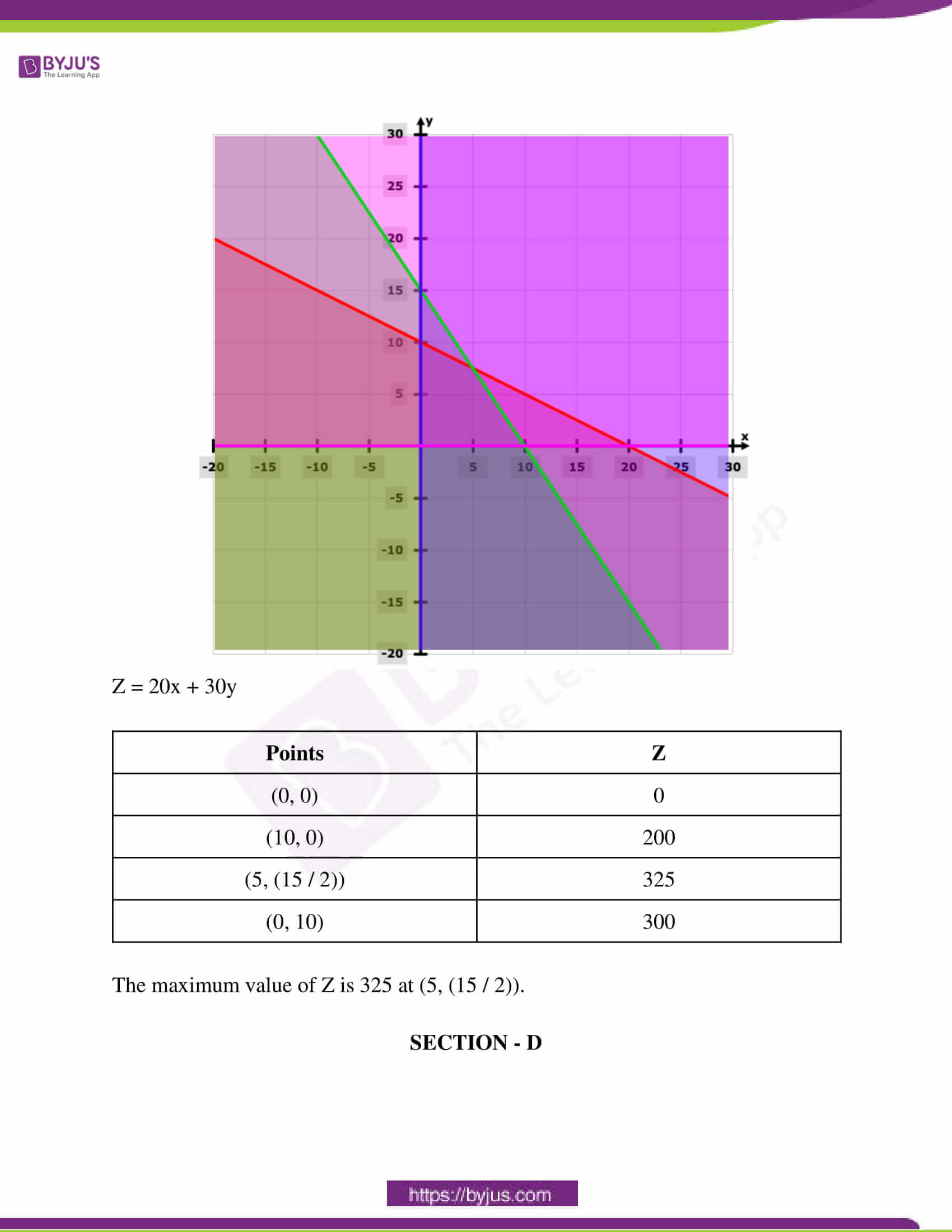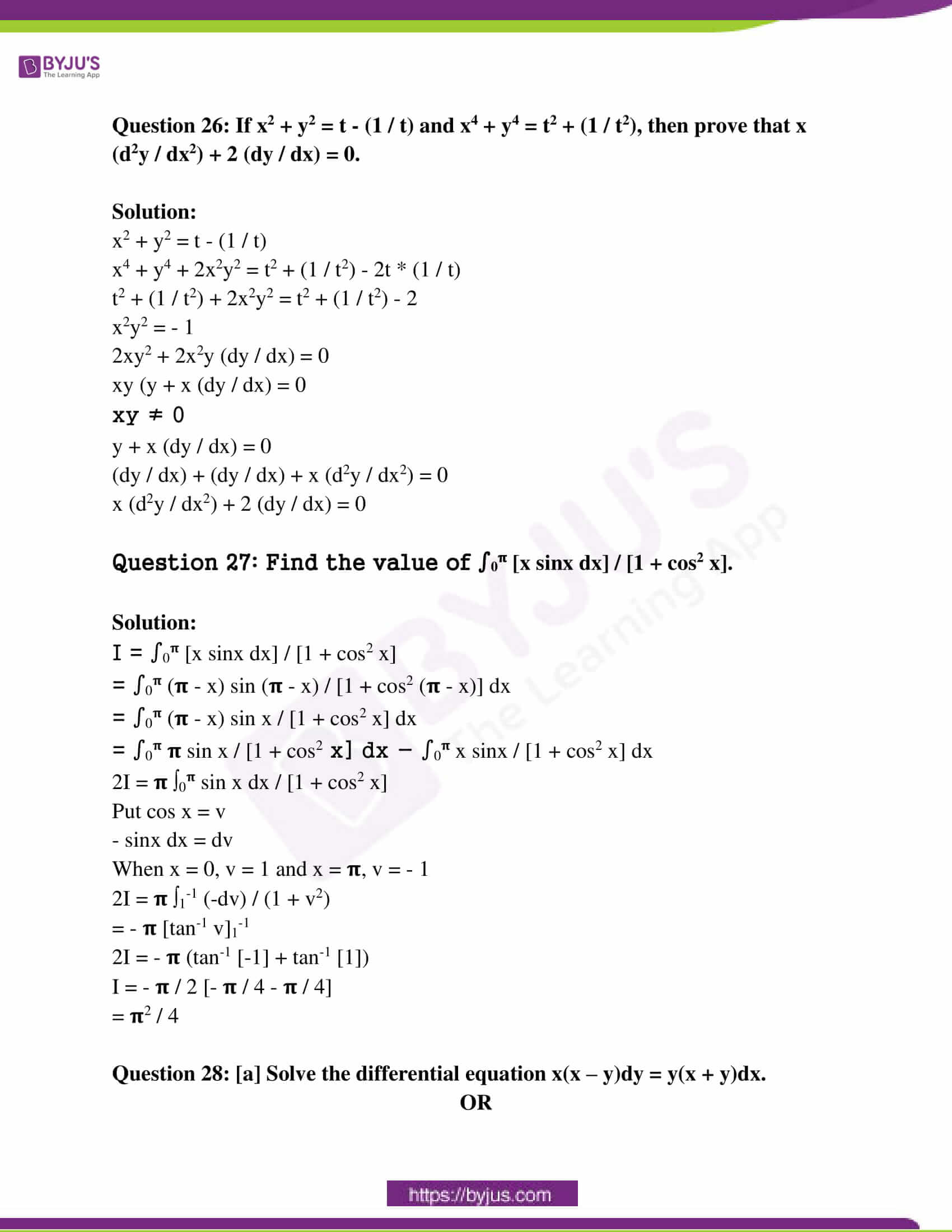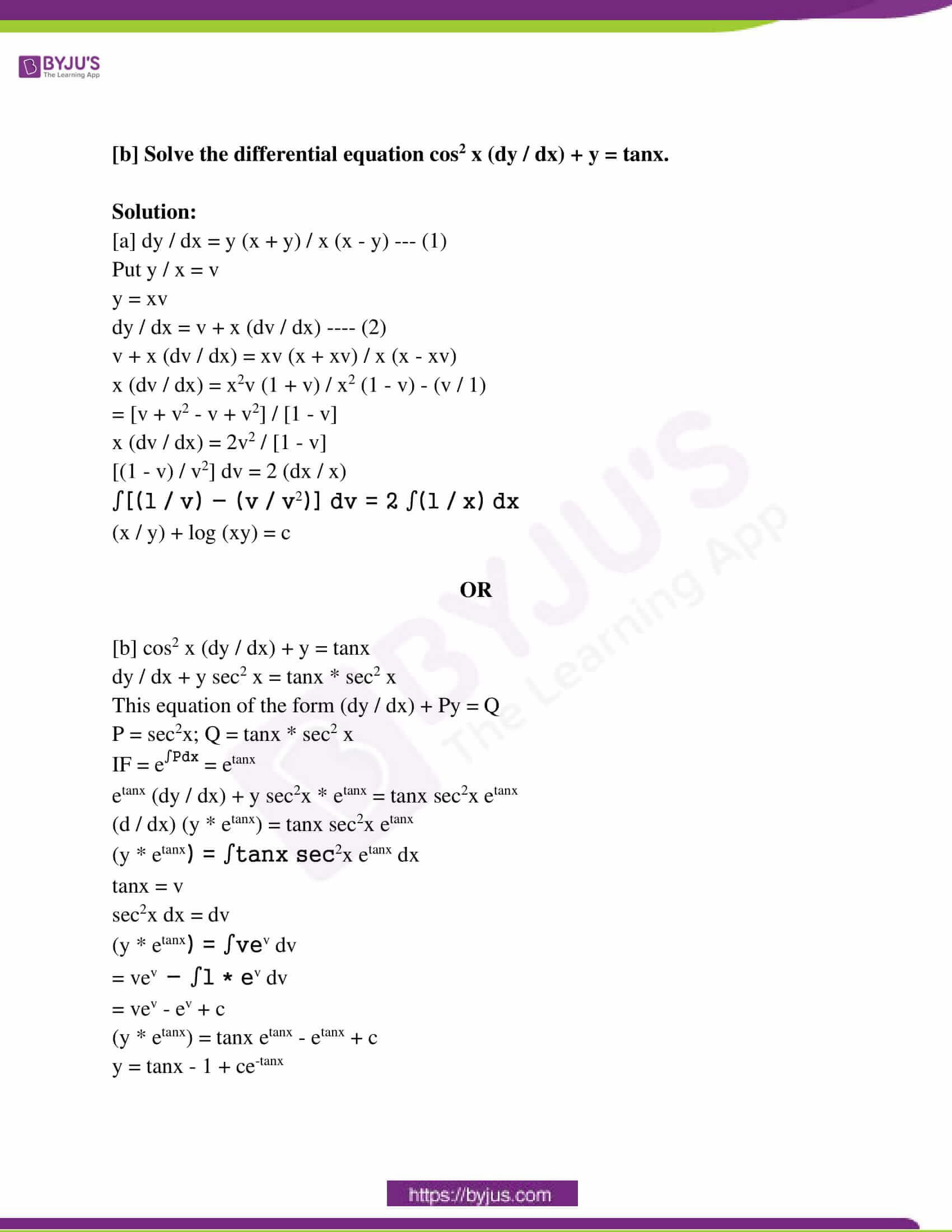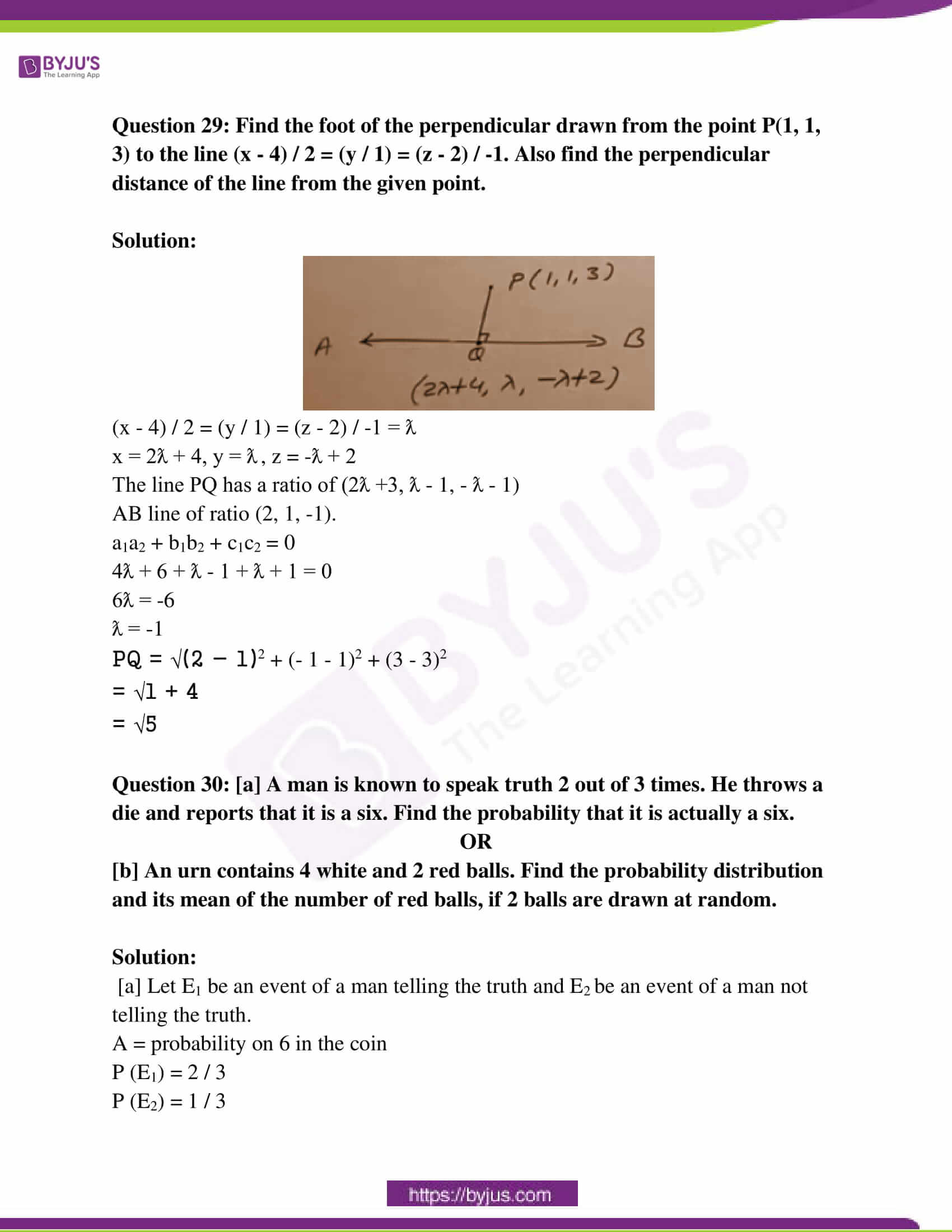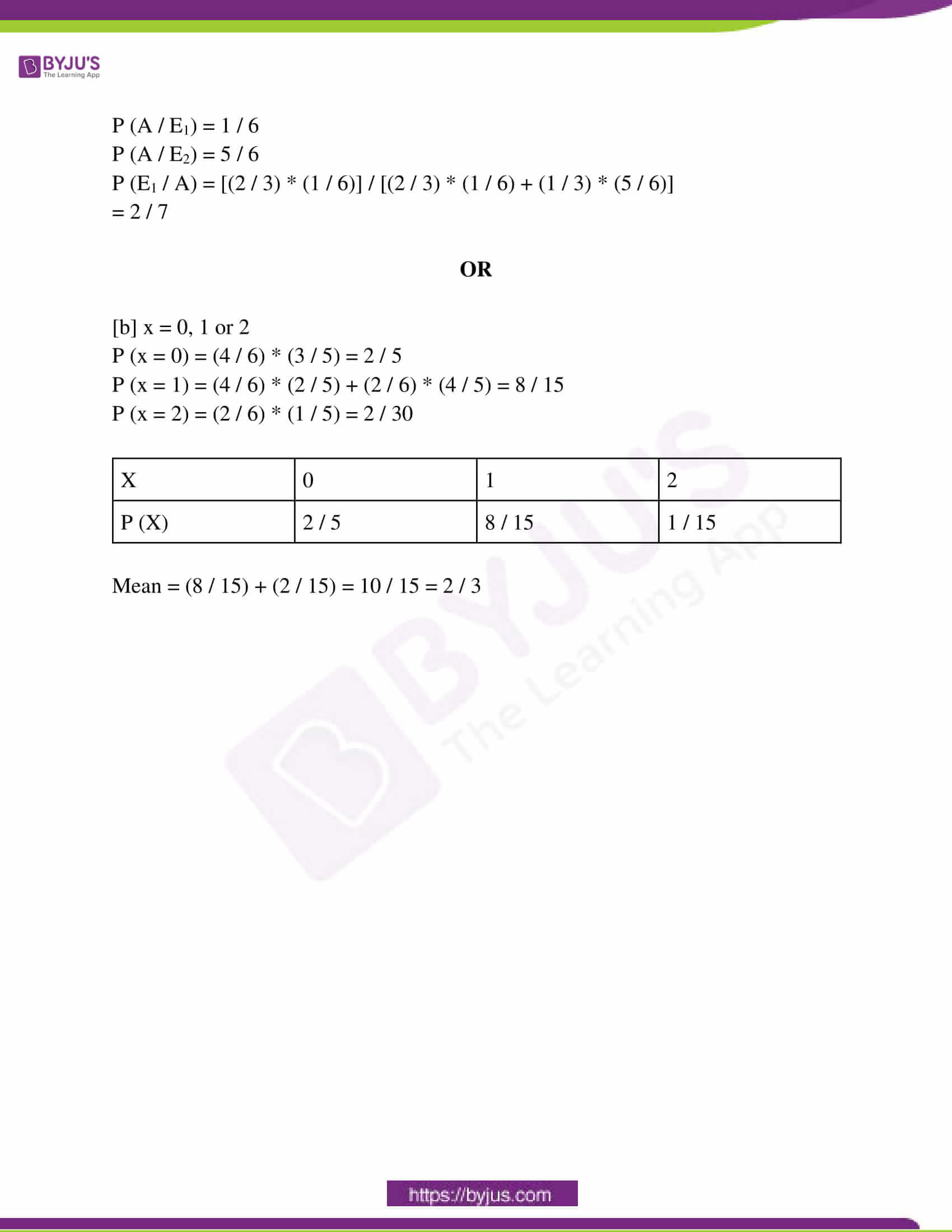SECTION – A

Question 1: If f : R → R, f (x) = sin x and g : R → R, g (x) = x2 then find g o f (x).

Solution:

f : R → R, f (x) = sin x

g : R → R, g (x) = x2

g o f (x) = g [ f (x)]

= g [sin x]2

g o f = sin2 x

Question 2: Find the value of sin [tan-1 (1) + cos-1 (1 / √2)].

Solution:

sin [tan-1 (1) + cos-1 (1 / √2)]

= sin [(𝛑 / 4) + (𝛑 / 4)]

= sin (2𝛑 / 4)

= sin (𝛑 / 2)

Question 3: If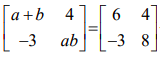, then find the value of a and b.

Solution:

Since the matrices are equal,

a + b = 6 —- (1)

ab = 8

a = 8 / b —- (2)

Put (2) in (1),

(8 / b) + (b) = 6

8 + b2 = 6b

b2 – 6b + 8 = 0

b2 – 4b – 2b + 8 = 0

b (b – 4) – 2 (b – 4) = 0

(b – 2) (b – 4) = 0

b = 2, 4

When b = 2, a = 4

When b = 4, a = 2

The value of a and b are 2 and 4, respectively.

Question 4: If matrix A =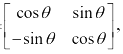then find the A-1.

Solution:

A-1 = adj A / |A|

|A| = cos2 θ + sin2 θ = 1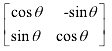A-1 =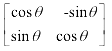/ 1

=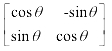Question 5: ∫(1 – cos 2x) / (1 + cos 2x) dx.

Solution:

= ∫(1 – cos 2x / 1 + cos 2x) dx

= ∫(2 sin2 x / 2 cos2 x) dx

= ∫tan2 x dx

= ∫(sec2 x – 1) dx (since tan2 x = sec2 x – 1)

= ∫sec2 x dx – ∫1 dx

= tanx – x + c (since ∫sec2 x dx = tan x)

Question 6: Find the angle between vectors 2i – j and i + 2j.

Solution:

Vector a = 2i – j

Vector b = i +2j

a . b = |a| |b| cos θ

(2i – j) (i + 2j) = √4 + 1 √(1 + 4) cos θ

(2 – 2) = √5 √5 cos θ

cos θ = 0

θ = 𝛑 / 2

Question 7: If |a| = 10, |b| = 2 and a . b = 12, then find the value of sin θ where θ is the angle between the vectors a and b.

Solution:

a . b = |a| |b| cos θ

12 = 10 * 2 cos θ

12 / 20 = cos θ

cos θ = 3 / 5

sin θ = √1 – cos2 θ

= √1 – (9 / 25)

= √16 / 25

= 4 / 5

Question 8: Find the direction cosines of the line passing through the points (1, 0, 0) and (0, 1, 1).

Solution:

Direction cosines are [1 / √(-1)2 + 12 + 12], [1 / √(-1)2 + 12 + 12], [1 / √(-1)2 + 12 + 12]

-1 / √3, 1 / √3 and 1 / √3.

Question 9: Show the region of the feasible solution under the following constraints.

2x + 3y ≤ 6, x ≥ 0, y ≥ 0.

Solution: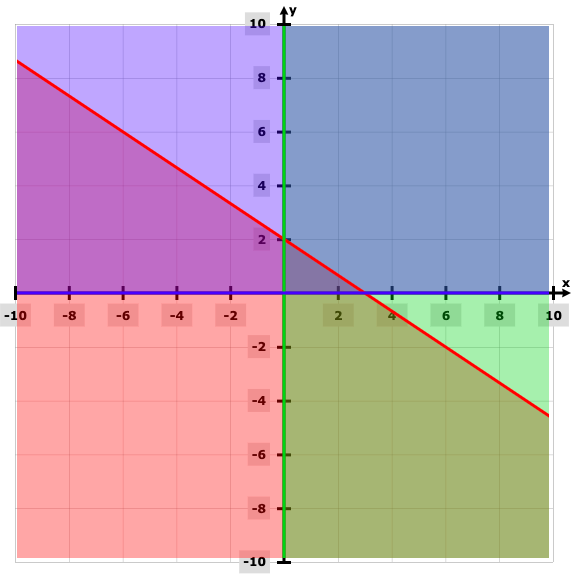Question 10: If P (A) = 0.6, P (B) = 0.3 and P (A ⋂ B) = 0.2, then find P (A / B).

Solution:

P (A) = 0.6

P (B) = 0.3

P (A ⋂ B) = 0.2

P (A / B) = P (A ⋂ B) / P (B)

= 0.2 / 0.3

= 2 / 3

P (A / B) = 0.67

SECTION – B

Question 11: If f (x) = (x – 3) / (x + 1), then find f [f (f (x) )].

Solution:

f (x) = (x – 3) / (x + 1)

f [f (f (x) )]

= f [f ((x – 3) / (x + 1))]

= f [{(x – 3) / (x + 1) – 3} / {(x – 3) / (x + 1) + 1}]

= f [(x – 3 – 3x -3) / (x – 3 + x + 1)]

= f [(-2x – 6) / (2x – 2)]

= f [(x + 3) / (1 – x)]

= [{(x – 3) / (1 – x) – 3} / {(x – 3) / (1 – x) + 1}]

= (x + 3 – 3 + 3x) / (x + 3 + 1 – x)

= 4x / 4

= x

Question 12: If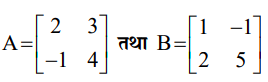, then prove that (AB)T = BTAT.

Solution: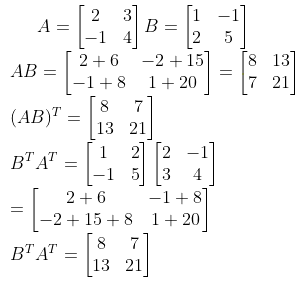So, (AB)T = BTAT.

Question 13: If function f (x) =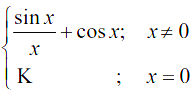is continuous at point x = 0, then find the value of K.

Solution:

On simplifying the expression, x = 0, value on the function, f (0) = k

x = 0 on the right hand side

= lim h→0 f (0 + h)

= lim h→0 (sin h / h + cos h)

= lim h→0 (sin h / h) + lim h→0 cos h

= 1 + 1

= 2

k = 2

Question 14: Find ∫1 / [cos2 (3x + 2)] dx.

Solution:

∫1 / [cos2 (3x + 2)] dx

= sec2 (3x + 2) dx

= (1 / 3) tan (3x + 2) + c

Put (3x + 2) = t

dt = 3 dx

dx = dt / 3

= ∫sec2 t (dt / 3)

= tan t / 3 + c

Question 15: If two sides of a triangle are represented by vectors i + 2j + 2k and 3i – 2j + k, then find the area of the triangle.

Solution:

a = i + 2j + 2k

b = 3i – 2j + k

a x b =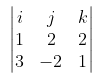= i (2 + 4) – j (1 – 6) + k (- 2 – 6)

= 6i + 5j – 8k

|a x b| = √36 + 25 + 64

= √125

= 5√5

The area of the triangle is 5√5 units.

SECTION – C

Question 16: [a] Prove that cos-1 (63 / 65) + 2 tan-1 (1 / 5) = sin-1 (3 / 5).

OR

[b] Solve the equation tan-1 3x + tan-1 2x = 𝛑 / 4.

Solution:

[a] LHS = cos-1 (63 / 65) + 2 tan-1 (1 / 5)

Take θ = cos-1 (63 / 65)

cos θ = 63 / 65

sin θ = √1 – cos2 θ

= √1 – (63 / 65)2

sin θ = 16 / 65

θ = sin-1 (16 / 65)

= sin-1 (16 / 65) + tan-1 (2 * (1 / 5)) / (1 – (1 / 25))

= sin-1 (16 / 65) + tan-1 (10 / (25 – 1))

= sin-1 (16 / 65) + tan-1 (5 / 12)

θ = tan-1 (5 / 12)

tan θ = 5 / 12

sec2 θ = 1 + tan2 θ

= 1 + (25 / 144)

= 169 / 144

sec θ = 13 / 12

cos θ = 12 / 13

sin θ = √1 – (12 / 13)2

= √1 – [144 / 169]

= √25 / 169

= 5 / 13

= sin-1 (16 / 65) + sin-1 (5 / 13)

sin-1 x + sin-1 y = sin-1 [x + √1 – y2 + y √1 – x2]

= sin-1 [(16 / 65) (√1 – (5 / 13)2) + (5 / 13) (√1 – (16 / 65)2)

= sin-1 ((16 / 65) * (12 / 13) + (5 / 13) * (63 / 65))

= sin-1 ([192 + 315] / (63 / 65))

= sin-1 [507 / (65 * 13)]

= sin-1 (3 / 5)

[b] tan-1 3x + tan-1 2x = 𝛑 / 4

tan-1 [3x + 2x / (1 – (3x) (2x))] = 𝛑 / 4

5x / [1 – 6x2] = 1

5x = 1 – 6x2

6x2 + 5x – 1 = 0

6x2 + 6x – x – 1 = 0

6x (x + 1) – 1 (x + 1) = 0

(x + 1) (6x – 1) = 0

x = -1 , 1 / 6

Question 17: Prove that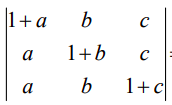= (1 + a + b + c).

Solution: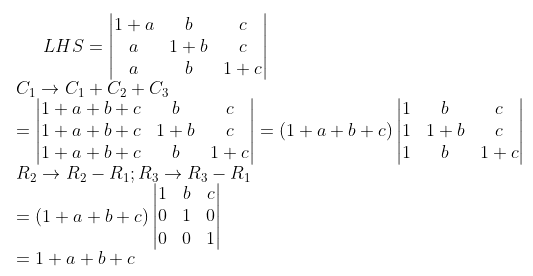Question 18: Solve the system of linear equations x + y + 2z = 0, x + 2y – z = 9, x – 3y + 3z = –14 by using the matrix method.

Solution: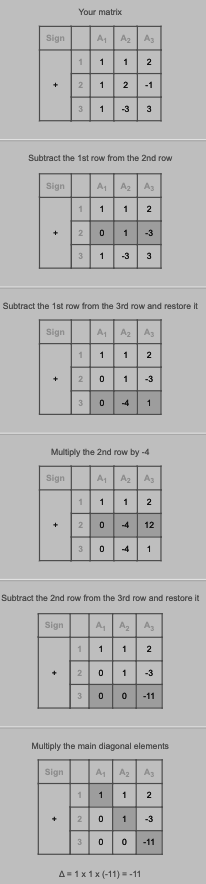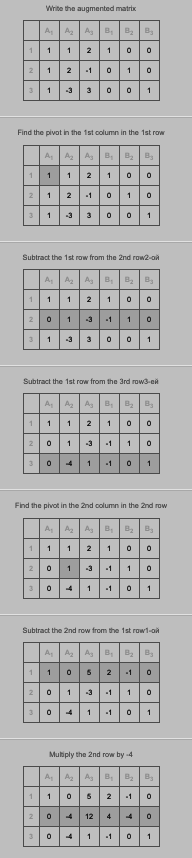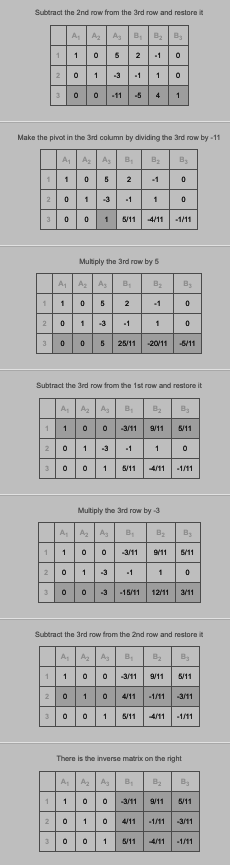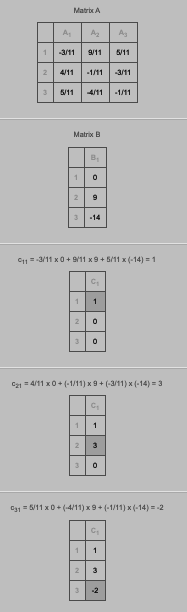Question 19: Find the equation of tangent of a curve y = x2 – 2x + 3 which is parallel to the line 2x – y + 9 = 0.

Solution:

y = mx + c

Slope of the tangent line = 2

dy / dx = 2x – 2

Therefore the tangent line of slope point in (x,y).

2x – 2 = 2

2x = 4

x = 2

y = 4 – 4 + 3 = 3

Slope = 2 at point (2, 3)

The tangent line equation is y – y1 = m (x – x1)

y – 3 = 2 (x – 2)

2x – y = 1

Question 20: If the radius of a sphere is measured as 7 cm with an error of 0.01 cm, then find the approximate error in calculating its volume.

Solution:

r = 7cm

Δr = 0.01 cm

V = (4 / 3) 𝛑r3

dv / dr = (4 / 3) 𝛑3r2

= 4𝛑r2

= 4𝛑 * 49

= 196 𝛑 cm3 / cm

Δv = [dv / dr] * (Δr)

Δv = [196 𝛑] * (0.01)

= 1.96 𝛑

Question 21: [a] Find ∫{cos x / √4 – sin2 x} dx.

OR

[b] Find ∫x tan-1 x dx.

Solution:

[a] ∫{cos x / √4 – sin2 x} dx

I = ∫{cos x / √4 – sin2 x} dx

Put sin x = v

cos x dx = dv

= ∫dv / √22 – v2

∫dx / √a2 – x2 = sin-1 (x / a) + c

I = sin-1 (v / 2) + c

= sin-1 (sin x / 2) + c

OR

[b] ∫x tan-1 x dx

I = ∫x tan-1 x dx

I = (x2 / 2) tan-1 x + ∫(x2 / 2) (1 / 1 + x2) dx

= (x2 / 2) tan-1 x + (1 / 2) ∫(x2 + 1 – 1) / (1 + x2) dx

= (x2 / 2) tan-1 x + (1 / 2) ∫(1 – [1 / 1 + x2]) dx

= (x2 / 2) tan-1 x – (1 / 2)x + (1 / 2) tan-1 x + c

Question 22: Find the area bounded by the parabola x2 = 4y and line y = 3. (Draw the figure in answer-book)

Solution: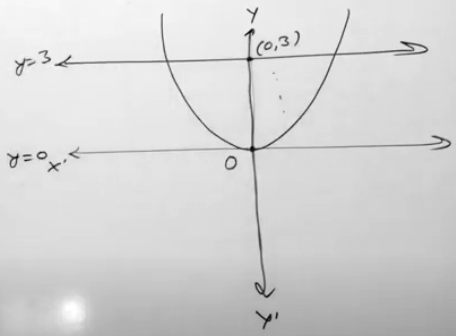2∫03 √4y dy

= 4 ∫03 y1/2 dy

= 4 [y1/2+1 / (1 / 2 + 1)]03

= 4 [33/2 / (3 / 2) – 0]

= 4 * (2 / 3) * (33/2)

= 8√3

Question 23: Find the area of the region given by: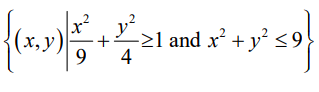.

Solution:

x2 / 32 + y2 / 22 ≥ 1

0 + 0 ≥ 1

x2 + y2 ≤ 3

0 + 0 ≤ 9

x2 + y2 = 9

y = √9 – x2

x2 / 32 + y2 / 22 = 1

y2 = (4 / 9) (9 – x2)

Area = 4 ∫03 √9 – x2 dx – 4 ∫03 (2 / 3) √9 – x2 dx

= (4 / 3) ∫03 √9 – x2 dx

= (4 / 3) [(x / 2) √9 – x2 + (9 / 2) sin-1 (x / 3)]03

= (4 / 3) [(9 / 2) * (𝛑 / 2)]

= 3𝛑

Question 24: [a] If a x b = c x d and a x c = b x d, then prove that a – d is parallel to b – c.

OR

[b] The four vertices of a tetrahedron are respectively O(0, 0, 0), A(1, 2, 1),

B(2, 1, 3) and C(1, 1, 2). Find the volume of the tetrahedron.

Solution:

[a] a x b = c x d —- (1)

a x c = b x d —- (2)

(a x b) – (a x c) = (c x d) – (b x d)

a x (b – c) = (c – b) x d

a x (b – c) – (c – b) x d = 0

a x (b – c) – d x (b – c) = 0

(a – d) (b – c) = 0

So, a – d is parallel to b – c.

OR

[b] O (0, 0, 0), A (1, 2, 1), B (2, 1, 3) and C (1, 1, 2).

a = i + 2j + k

b = 2i + j + 3k

c = i + j + 2k

Volume = (1 / 6) [a b c]

= (1 / 6)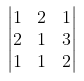= (1 / 6) [1 (2 – 3) – 2 (4 – 3) + 1 (2 – 1)]

= (1 / 6) [- 1 – 2 + 1]

= – 1 / 3

= 1 / 3 unit3

Question 25: By the graphical method, solve the following linear programming problem for

Maximize Z = 20x + 30y

Constraints

x + 2y ≤ 20

3x + 2y ≤ 30

x ≥ 0, y ≥ 0.

Solution: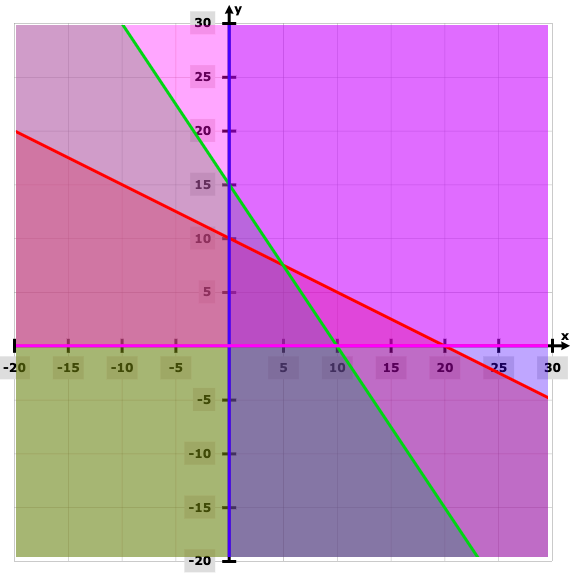Z = 20x + 30y

 Points Z (0, 0) 0 (10, 0) 200 (5, (15 / 2)) 325 (0, 10) 300

The maximum value of Z is 325 at (5, (15 / 2)).

SECTION – D

Question 26: If x2 + y2 = t – (1 / t) and x4 + y4 = t2 + (1 / t2), then prove that x (d2y / dx2) + 2 (dy / dx) = 0.

Solution:

x2 + y2 = t – (1 / t)

x4 + y4 + 2x2y2 = t2 + (1 / t2) – 2t * (1 / t)

t2 + (1 / t2) + 2x2y2 = t2 + (1 / t2) – 2

x2y2 = – 1

2xy2 + 2x2y (dy / dx) = 0

xy (y + x (dy / dx) = 0

xy ≠ 0

y + x (dy / dx) = 0

(dy / dx) + (dy / dx) + x (d2y / dx2) = 0

x (d2y / dx2) + 2 (dy / dx) = 0

Question 27: Find the value of ∫0𝛑 [x sinx dx] / [1 + cos2 x].

Solution:

I = ∫0𝛑 [x sinx dx] / [1 + cos2 x]

= ∫0𝛑 (𝛑 – x) sin (𝛑 – x) / [1 + cos2 (𝛑 – x)] dx

= ∫0𝛑 (𝛑 – x) sin x / [1 + cos2 x] dx

= ∫0𝛑 𝛑 sin x / [1 + cos2 x] dx – ∫0𝛑 x sinx / [1 + cos2 x] dx

2I = 𝛑 ∫0𝛑 sin x dx / [1 + cos2 x]

Put cos x = v

– sinx dx = dv

When x = 0, v = 1 and x = 𝛑, v = – 1

2I = 𝛑 ∫1-1 (-dv) / (1 + v2)

= – 𝛑 [tan-1 v]1-1

2I = – 𝛑 (tan-1 [-1] + tan-1 )

I = – 𝛑 / 2 [- 𝛑 / 4 – 𝛑 / 4]

= 𝛑2 / 4

Question 28: [a] Solve the differential equation x(x – y)dy = y(x + y)dx.

OR

[b] Solve the differential equation cos2 x (dy / dx) + y = tanx.

Solution:

[a] dy / dx = y (x + y) / x (x – y) — (1)

Put y / x = v

y = xv

dy / dx = v + x (dv / dx) —- (2)

v + x (dv / dx) = xv (x + xv) / x (x – xv)

x (dv / dx) = x2v (1 + v) / x2 (1 – v) – (v / 1)

= [v + v2 – v + v2] / [1 – v]

x (dv / dx) = 2v2 / [1 – v] [(1 – v) / v2] dv = 2 (dx / x)

∫[(1 / v) – (v / v2)] dv = 2 ∫(1 / x) dx

(x / y) + log (xy) = c

OR

[b] cos2 x (dy / dx) + y = tanx

dy / dx + y sec2 x = tanx * sec2 x

This equation of the form (dy / dx) + Py = Q

P = sec2x; Q = tanx * sec2 x

IF = e∫Pdx = etanx

etanx (dy / dx) + y sec2x * etanx = tanx sec2x etanx

(d / dx) (y * etanx) = tanx sec2x etanx

(y * etanx) = ∫tanx sec2x etanx dx

tanx = v

sec2x dx = dv

(y * etanx) = ∫vev dv

= vev – ∫1 * ev dv

= vev – ev + c

(y * etanx) = tanx etanx – etanx + c

y = tanx – 1 + ce-tanx

Question 29: Find the foot of the perpendicular drawn from the point P(1, 1, 3) to the line (x – 4) / 2 = (y / 1) = (z – 2) / -1. Also find the perpendicular distance of the line from the given point.

Solution: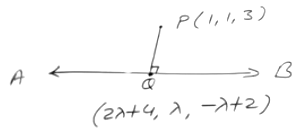(x – 4) / 2 = (y / 1) = (z – 2) / -1 = ƛ

x = 2ƛ + 4, y = ƛ , z = -ƛ + 2

The line PQ has a ratio of (2ƛ +3, ƛ – 1, – ƛ – 1)

AB line of ratio (2, 1, -1).

a1a2 + b1b2 + c1c2 = 0

4ƛ + 6 + ƛ – 1 + ƛ + 1 = 0

6ƛ = -6

ƛ = -1

PQ = √(2 – 1)2 + (- 1 – 1)2 + (3 – 3)2

= √1 + 4

= √5

Question 30: [a] A man is known to speak truth 2 out of 3 times. He throws a die and reports that it is a six. Find the probability that it is actually a six.

OR

[b] An urn contains 4 white and 2 red balls. Find the probability distribution and its mean of the number of red balls, if 2 balls are drawn at random.

Solution:

[a] Let E1 be an event of a man telling the truth and E2 be an event of a man not telling the truth.

A = probability on 6 in the coin

P (E1) = 2 / 3

P (E2) = 1 / 3

P (A / E1) = 1 / 6

P (A / E2) = 5 / 6

P (E1 / A) = [(2 / 3) * (1 / 6)] / [(2 / 3) * (1 / 6) + (1 / 3) * (5 / 6)]

= 2 / 7

OR

[b] x = 0, 1 or 2

P (x = 0) = (4 / 6) * (3 / 5) = 2 / 5

P (x = 1) = (4 / 6) * (2 / 5) + (2 / 6) * (4 / 5) = 8 / 15

P (x = 2) = (2 / 6) * (1 / 5) = 2 / 30

 X 0 1 2 P (X) 2 / 5 8 / 15 1 / 15

Mean = (8 / 15) + (2 / 15) = 10 / 15 = 2 / 3